# Houseplant CTF 2020 Writeups

## JS Lotto

I liked the concept for this challenge, but unfortunately a script to solve this exact problem already existed online. I took first blood on this challenge though, which I was pretty happy about.

Opening the website we see a webpage that asks us to input five numbers from 0 to 1000.Opening the source code of the we site, we see a `app.js` which sends and recieves requests from the server. In the code there are references to `Math.random()`, which makes me think that the backend server is also generating its numbers this way.

Doing some research into predicting `Math.random()`, we find this page, which talks about using a script to predict virual Powerball lottery numbers generated in JS. Hmmm…

They provide a link to a GitHub with a Python script that uses Z3 to solve for the state.

I edited the script to connect it to the `/guess` endpoint. First, the script requests for 5 numbers, turns them into `Math.random()` floats, then solves for the state using Z3. Then, the next 500 numbers are generated. A second request is sent to get 5 more numbers to figure out where in my predicted numbers the server currently is. Finally, the next 5 numbers after that point are the answer sent to the server, which gives the flag!

``````import sys
import math
import struct
import random
from z3 import *
import requests

def xs128p(state0, state1):
s1 ^= (s1 << 23) & MASK
s1 ^= (s1 >> 17) & MASK
s1 ^= (s0 >> 26) & MASK

return state0, state1, generated

def sym_xs128p(slvr, sym_state0, sym_state1, generated):
s1 = sym_state0
s0 = sym_state1
s1 ^= (s1 << 23)
s1 ^= LShR(s1, 17)
s1 ^= s0
s1 ^= LShR(s0, 26)
sym_state0 = sym_state1
sym_state1 = s1
calc = sym_state0

condition = Bool('c%d' % int(generated * random.random()))
impl = Implies(condition, LShR(calc, 12) == int(generated))

return sym_state0, sym_state1, [condition]

def reverse17(val):
return val ^ (val >> 17) ^ (val >> 34) ^ (val >> 51)

def reverse23(val):
return (val ^ (val << 23) ^ (val << 46)) & MASK

def xs128p_backward(state0, state1):
prev_state1 = state0
prev_state0 = state1 ^ (state0 >> 26)
prev_state0 = prev_state0 ^ state0
prev_state0 = reverse17(prev_state0)
prev_state0 = reverse23(prev_state0)
generated = prev_state0

return prev_state0, prev_state1, generated

def to_double(out):
double_bits = (out >> 12) | 0x3FF0000000000000
double = struct.unpack('d', struct.pack('<Q', double_bits)) - 1
return double

def main():
req = requests.post("http://challs.houseplant.riceteacatpanda.wtf:30006/guess", json=[1,2,3,4,5])
dubs = []
for v in req.json()['results']:
dubs.append(v/1000)
print('initial numbers', dubs)

dubs = dubs[::-1]
generated = []
for idx in range(len(dubs)):
recovered = struct.unpack('<Q', struct.pack('d', dubs[idx] + 1)) & (MASK >> 12)
generated.append(recovered)

ostate0, ostate1 = BitVecs('ostate0 ostate1', 64)
sym_state0 = ostate0
sym_state1 = ostate1
slvr = Solver()
conditions = []

for ea in range(len(dubs)):
sym_state0, sym_state1, ret_conditions = sym_xs128p(slvr, sym_state0, sym_state1, generated[ea])
conditions += ret_conditions

if slvr.check(conditions) == sat:
m = slvr.model()
state0 = m[ostate0].as_long()
state1 = m[ostate1].as_long()
slvr.add(Or(ostate0 != m[ostate0], ostate1 != m[ostate1]))
if slvr.check(conditions) == sat:
print('WARNING: multiple solutions found! use more dubs!')

generated = []
for idx in range(500):
state0, state1, out = xs128p_backward(state0, state1)

double = to_double(out)
generated.append(double)

req2 = requests.post("http://challs.houseplant.riceteacatpanda.wtf:30006/guess", json=[1,2,3,4,5])
dubs2 = []
for v in req2.json()['results']:
dubs2.append(v/1000)
print('marker numbers', dubs2)

last = dubs2[-1]
start = generated.index(last)

print(final.text)
else:
print('unsolvable')

main()
``````

Flag: rtcp{th3_h0us3_d1dnt_w1n_th15_t1m3_5bcbf4}

Adventure-Revisited was a pwn challenge in this CTF, but I thought it was more of a misc challenge.

You’re directed to #adventure-revisited on the CTF discord, and going there shows me a Discord bot. To solve the challenge, you need to pwn the bot to give you a flag.

Checking the chat, some people ran the command `jst eval` on the bot, but it errored out and said that you could not eval in this guild.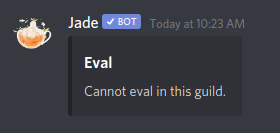So, I invited the bot onto a private test Discord server, and tried out `jst eval` again.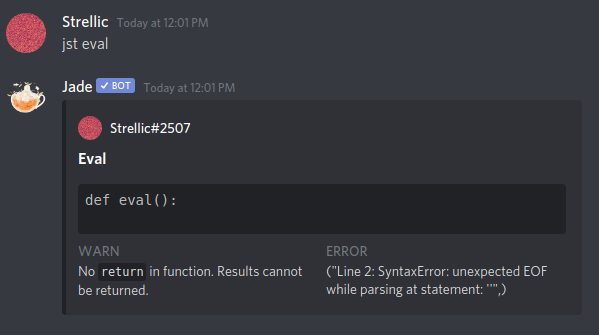`jst eval` runs Python code! I immediately tried to get some sort of a shell, but unfortunately, import, exec, eval, and even the double-underscore tricks are not allowed. Next, I hoped to try and see if the flag was stored in a global variable, so I ran `jst eval return globals()`, which returned this.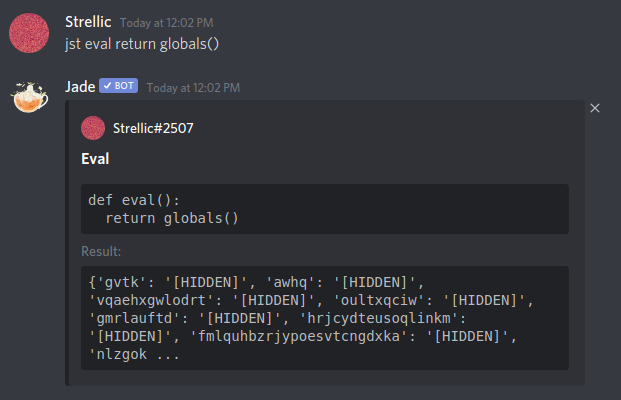The return value has a limited size, so the massive amount of random garbage in `globals()` hides any important data. However, using some Python list comprehension, we can filter all of the `[HIDDEN]`s out.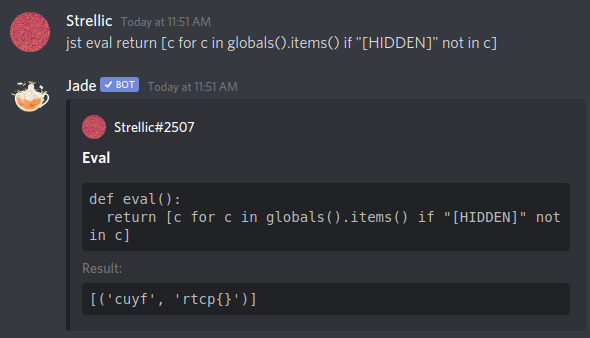This just leaves us with one value, `cuyf`, which has a blank flag. Huh. After like 30 more minutes of debugging and trying random things, I eventually did `jst eval return cuyf` and got the flag.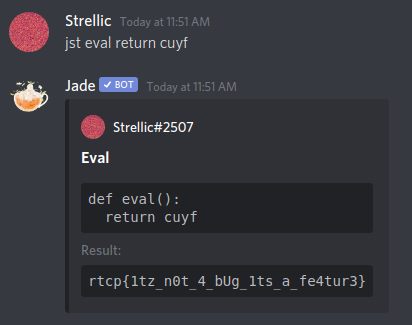## RTCP Trivia

This was an Android reversing challenge. The app connected via websockets to a server, which sent back and forth data to answer questions. To get the flag, you would need to answer 1000 questions correctly.

First thing I did was upload the .apk to http://www.javadecompilers.com/apk, which decompiled the apk and gave me some readable Java source.

Opening `Game.java`, we see “AES/CBC/PKCS7Padding”, which means that the server and client probably communicate through AES. Thankfully, the code to generate the key and IV has to be on the client somewhere, so if we can grab those values, we can use them to decrypt all the traffic.

To view the traffic, I used an Android emulator on my computer, and used Wireshark to inspect packets. Upon connecting to the server, a WebSocket connection is established to `ws://challs.houseplant.riceteacatpanda.wtf:40001`. All of the packets sent back and forth are bits of JSON data with a key named “method”, which describes what type of packet.

First, an `ident` packet is sent, with a `userToken` that is generated by the client. The server sends back an `ident` packet with `success` set to true, which lets the user know it is connected. Then the user and server send back and forth a `start` packet to start the game. Once the game is started, the server will send a `question` packet with a question `id`, `requestIdentifier`, `questionText`, `options`, and `correctAnswer`. However, `questionText, `options`, and `correctAnswer` are all encrypted using AES CBC. Since the client can still read the question, the app must have the AES key and IV somewhere.

Checking the decompiled `Game.java`, we see this:

``````JSONObject jSONObject = new JSONObject(this.f3310d);
String a = new C0784nx(Game.this.getIntent().getStringExtra("id"), Game.this.getResources()).mo2708a();
String string = jSONObject.getString("id");
StringBuilder sb = new StringBuilder();
sb.append(a);
sb.append(":");
sb.append(string);
byte[] a2 = C0784nx.m2603a(sb.toString());
byte[] b = C0784nx.m2604b(jSONObject.getString("requestIdentifier"));
SecretKeySpec secretKeySpec = new SecretKeySpec(a2, "AES");
IvParameterSpec ivParameterSpec = new IvParameterSpec(b);
``````

The game generates a new key and IV on every question. It generates the `secretKeySpec` key based on the user’s provided token and the id, but also used some methods in `C0784nx`, which I had to reverse next.

In `C0784nx.java`, we have methods that manipulate the data in some way to generate the key and IV. First, let’s look at the key.

The key is generated using the `mo2708a` method, which also uses the `m2605c` method.

``````private String m2605c(String str) {
MessageDigest instance = MessageDigest.getInstance("SHA-256");
StringBuilder sb = new StringBuilder();
sb.append(str);
sb.append(":");
sb.append(this.f3315b);
byte[] digest = instance.digest(new String(sb.toString()).getBytes());
StringBuffer stringBuffer = new StringBuffer();
for (byte b : digest) {
String hexString = Integer.toHexString(255 & b);
if (hexString.length() == 1) {
stringBuffer.append('0');
}
stringBuffer.append(hexString);
}
return String.valueOf(stringBuffer);
}

/* renamed from: a */
public final String mo2708a() {
InputStream openRawResource = this.f3314a.openRawResource(R.raw.correct);
byte[] bArr = new byte[openRawResource.available()];
byte[] bArr2 = new byte[openRawResource.available()];
openRawResource.close();
new ArrayList();
for (int i = 0; i < bArr.length; i++) {
double d = (double) i;
if (Math.sqrt(d) % 1.0d == 0.0d) {
bArr2[(int) Math.sqrt(d)] = bArr[i];
}
}
byte[] digest = MessageDigest.getInstance("SHA-256").digest(bArr2);
StringBuffer stringBuffer = new StringBuffer();
for (byte b : digest) {
String hexString = Integer.toHexString(255 & b);
if (hexString.length() == 1) {
stringBuffer.append('0');
}
stringBuffer.append(hexString);
}
return m2605c(String.valueOf(stringBuffer));
}
``````

Because of the `openRawResource` call, I couldn’t exactly reverse the function based on the Java alone. So, I used `apktool` to decompile the .apk into `.smali` code, and added Android log statements into the code. I then read the values printed to the log using `adb`.

Doing this led me to the realization that `stringBuffer` in `mo2708a` was the same every time, specifically “cbce23dfcdc7efe826d23bbf3d635d8fd55b6499d16ca8830a973ff57175119f”. From there, I just had to reverse `m2605c("cbce23dfcdc7efe826d23bbf3d635d8fd55b6499d16ca8830a973ff57175119f")`.

`m2605c` obviously had some SHA-256 magic, but after that was some weird `Integer.tohexString` stuff. However, after doing the math, I realized that the SHA-256 hash did nothing. Huh. So, `m2605c` just returns the SHA-256 hash of the parameter, which we know, and `this.f3315b`, which is the userToken.

Now that that is reversed, we can go back to `Game.java`. After this call, the result of this SHA-256 hash is run through `m2603a` with the question id, which just turns out to be another SHA-256 hash.

So, the formula for the key is sha256(sha256(cbce23dfcdc7efe826d23bbf3d635d8fd55b6499d16ca8830a973ff57175119f:userToken):id)).

Now, to figure out the IV, I just logged the IV as it was passed into `IvParameterSpec`, and it turned out just to be the `requestIdentifier` given by data.

Now that both the key and IV are obtained, we can now decrypt the packets sent by the server! `questionText` and `options` are the questions and answers respectively, and `correctAnswer` is the number of the correct answer choice! Knowing this, we can assemble a Python script which will run through all 1000 questions.

``````import json
import base64
import asyncio
import websockets
import hashlib
import binascii

from Crypto.Cipher import AES

BS = 16
return s + (BS - len(s) % BS) * chr(BS - len(s) % BS)
return s[0:-s[-1]]

class AESCipher:
def __init__( self, key, iv):
self.key = key
self.iv = iv

def encrypt( self, raw ):
cipher = AES.new( self.key, AES.MODE_CBC, self.iv )
return base64.b64encode( self.iv + cipher.encrypt( raw ) )

def decrypt( self, enc ):
enc = base64.b64decode(enc)
cipher = AES.new(self.key, AES.MODE_CBC, self.iv )

url = "ws://challs.houseplant.riceteacatpanda.wtf:40001"
userToken = "58e6384234e53bb09799556a2df8443ee4f0cb3274db5800855026c3ca2d3e4a"

def sha256(plaintext):
m = hashlib.sha256()
m.update(plaintext.encode("utf-8"))
return m.hexdigest()

async def run():
question = 1

async with websockets.connect(url) as websocket:
await websocket.send(json.dumps({
"method": "ident",
"userToken": userToken
}))

if resp["method"] == "ident" and resp["success"] == True:
print("Logged in successfully!")
print("Starting the game...")

await websocket.send(json.dumps({
"method": "start"
}))

if resp["method"] == "start" and resp["success"] == True:
print("The game has started!")

while True:
if data["method"] == "question":
id = data["id"]
iv = data["requestIdentifier"]
questionText = data["questionText"]

k1 = sha256(f"cbce23dfcdc7efe826d23bbf3d635d8fd55b6499d16ca8830a973ff57175119f:{userToken}")
key = sha256(f"{k1}:{id}")

cipher = AESCipher(bytes.fromhex(key), bytes.fromhex(iv))
print(f"Question {question}: {cipher.decrypt(questionText).decode('utf-8')}")

options = [cipher.decrypt(o).decode("utf-8") for o in data["options"]]
for choice in options:
print(f"\t{choice}")

await websocket.send(json.dumps({
}))

print("\n----\n")

question += 1
else:
print(data)
break

asyncio.get_event_loop().run_until_complete(run())
``````

Flag: rtcp{qu1z_4pps_4re_c00l_aeecfa13}